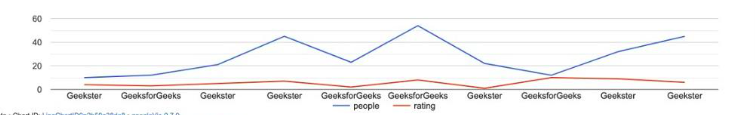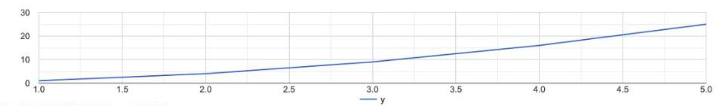Open in App
Not now

# How to Add Horizontal Discrete Legend Below the Chart in Ggvis

• Last Updated : 11 Oct, 2022

In this article, we are going to see how to add horizontal discrete legend below the chart in ggvis in R Programming Language.

### Add Horizontal Discrete Legend Below the Chart in Ggvis

The ggvis package in R is used for data visualization. It is also used to create interactive web graphics. It is also used to create interactive graphs and plots. It is an addition to the ggplot package. It provides the framework to build HTML graphs in the working space. The package can be downloaded and installed into the working space using the following command :

`install.packages("ggvis")`

A data frame can be created using the data.frame method which takes as input a number of columns and the values stored in it. This data can be visualized using the ggvis method which computes the visual representation of the y-axis  and x-axis data contained in the data frame. The ggvis method has the following syntax.

Syntax: ggvis(~y,~x,stroke)

Arguments:

• ~y,~x- y-axis and x-axis of the data frame
• stroke- the different color values to plot different lines in ggvis

The gvisLineChart is used to plot Google Line Chart with R. On plotting the data, a window screen opens plotting the data.

Syntax: gvisLineChart(data, options = list())

Arguments :

• data – The data frame to be plotted
• options – The specification for the data

## R

 `# installing the required libraries``library``(googleVis)``library``(tidyverse)` `# creating a data frame``data_frame = ``data.frame``(group = ``c``(``"Geekster"``,``"GeeksforGeeks"``,``                                  ``"Geekster"``,``"Geekster"``,``                                  ``"GeeksforGeeks"``,``"GeeksforGeeks"``,``                                  ``"Geekster"``,``"GeeksforGeeks"``,``                                      ``"Geekster"``,``"Geekster"``),``                        ``people = ``c``(10,12,21,45,23,54,``                                   ``22,12,32,45),``                        ``rating = ``c``(4,3,5,7,2,8,1,``                                   ``10,9,6))``# printing the data frame``print``(``"Original Data frame"``)``print``(data_frame)` `# creating a line chart for the data``graph <- ``gvisLineChart``(data_frame, options=``list``(``  ``legend=``"bottom"``))` `# plotting the data``plot``(graph)`

Output

``` "Original Data frame"
> print(data_frame)
group people rating
1       Geekster     10      4
2  GeeksforGeeks     12      3
3       Geekster     21      5
4       Geekster     45      7
5  GeeksforGeeks     23      2
6  GeeksforGeeks     54      8
7       Geekster     22      1
8  GeeksforGeeks     12     10
9       Geekster     32      9
10      Geekster     45      6```The data can also be plotted taking into consideration the functions. For instance, the line can be drawn for x and x^2 respectively.

## R

 `# installing the required libraries``library``(googleVis)``library``(tidyverse)` `# creating a data frame``data_frame = ``data.frame``(x = ``c``(1,2,3,4,5),``                        ``y = x^2``                        ``)``# printing the data frame``print``(``"Original Data frame"``)``print``(data_frame)` `# creating a line chart for the data``graph <- ``gvisLineChart``(data_frame, options=``list``(``  ``legend=``"bottom"``))``# plotting the data``plot``(graph)`

Output

``` "Original Data frame"
> print(data_frame)
x  y
1 1  1
2 2  4
3 3  9
4 4 16
5 5 25```My Personal Notes arrow_drop_up